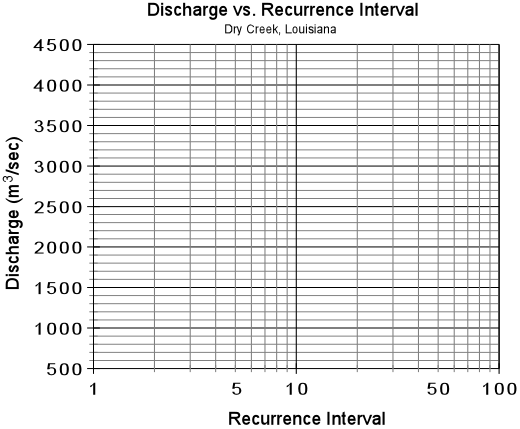EENS 3050 Natural Disasters Tulane University Prof. Stephen A. Nelson Homework Assignment IV. Flooding Exercises

 Flood frequency information can be determined from knowledge of the peak discharge (highest discharge) in any given year provided enough years of information has been collected.  This allows one to relate the expected recurrence interval for a given discharge, and determine the probability that a flood of a given discharge will occur in any given year.  The recurrence interval for a given discharge can be calculated by first ranking the discharges.  In the table below for Dry Creek, Louisiana, fill in the Rank column.  To do this, enter a 1 for the maximum discharge that has occurred during the 20 years of available data. The second highest discharge will be given a rank of 2, etc. with the lowest discharge given a value of 20. (1 point)

 Date Discharge (m3/sec) Rank, m Recurrence Interval,R 13-Mar-97 750 06-Mar-98 1250 28-Feb-99 1410 04-Mar-00 2890 22-Mar-01 1850 03-Mar-02 800 12-Mar-03 1000 01-Feb-04 692 04-Apr-05 1350 02-May-06 1200 16-Mar-07 850 06-Jul-08 2400 21-Feb-09 890 30-Jan-10 1480 16-Mar-11 1500 21-Feb-12 1300 12-May-13 1700 08-Apr-14 2200 01-Mar-15 1830 08-Feb-16 1120
 After you have filled in the Rank column, you can now calculate the recurrence interval for each peak discharge.  The recurrence interval, R, is given by the Weibull Equation:   R = (n+1)/m where n is the number of years over which the data was collected (20 years in this case) and m is the rank of each peak discharge.  Use this equation to calculate the recurrence interval for each peak discharge. (2 points) Next, use the graph below to plot a graph of discharge (on the y-axis) versus recurrence interval (on the x-axis).  Note that the x-axis is a logarithmic scale, and thus you should try to estimate as best you can where the data point will fall between the lines on the graph. Once you have plotted the points use a ruler to draw the best fit straight line through the data points (lay a ruler on the graph and try to draw a line that most closely approximates all of the data points). Do not draw lines that connect individual data points.  Make sure you turn in the completed table above and the graph below. (2 points)By extrapolating your line on the graph, determine the peak discharge expected in a flood with a recurrence interval of 50 years and 100 years.  These are the discharges expected in a 50 year flood and a 100 year flood. (1 point) From reading of your text and lecture notes, is it possible that discharges associated with a 100 year flood could occur 5 years apart, 10 years apart, or even one year apart? (0.5 point) The annual exceedence probability, Pe, is the probability that a given discharge will occur in a given year.  It is calculated as the inverse of the recurrence interval, R: Pe = 1/R Thus, the probability that a flood with a ten year recurrence interval will occur in any year is 1/10 = 0.1 or 10%.  What are the probabilities that a 50 year flood and a 100 year flood will occur in any given year? (1 point)   The mean annual discharge can be calculated by summing the peak annual discharges and dividing by the number of years.  What is the mean annual discharge for Dry Creek? (0.5 point) Flood stage, or bankfull stage on Dry Creek occurs at a discharge of 1600 m3/sec. What is the recurrence interval of such a discharge according to your graph? (1 point) Someone has offered to sell you a 4-bedroom 2-story house with a 2 car garage and swimming pool on a 1 acre lot on a relatively flat piece of ground on the banks of Dry Creek for what seems like a reasonable price of \$75,000.  The last time the house was flooded it cost \$56,000 to repair the flood damage.  How often has the house been flooded in the last 20 years?  What is the probability that the house will be flooded in the first year that you own it?  Would you still consider buying the house?   Why or why not? (2 points)